# Time

Knowing the concept of time is very essential in planning our day-to-day activities. It helps us to plan our tasks and makes us more efficient organizers. In this lesson, we will learn about the basics of time, the history and origin of time, time meaning, measurement of time in math, units of time, how to read time and also weeks, months and years.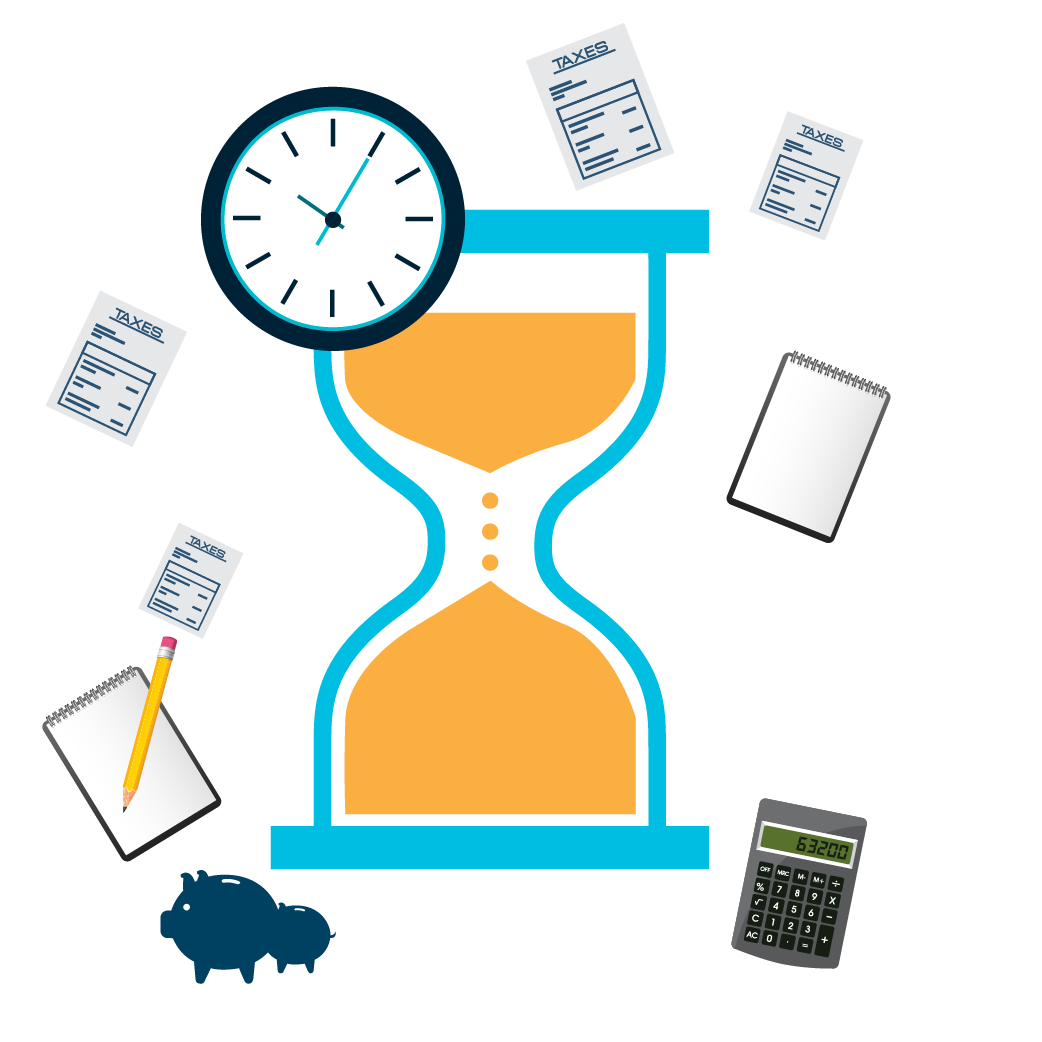## Lesson Plan

 1 What Is Time? 2 Important Notes on Time 3 Solved Examples on Time 4 Interactive Questions on Time 5 Challenging Questions on Time

Introduction to the History of Time

Time never stops or rests. It is measurable only once and is infinite. As the Earth rotates on its axis we experience day and night and as the it revolves around the Sun we experience various seasons and months of the year. To be precise, we can say that time is represented by change.

According to the big bang theory, our Universe emerged billions of years ago and that is when time started.

Let us now get in to the world of time and start exploring it!

## What Is Time?

### Time Definition

We can define time as a period during which a certain event happens, has happened or is going to happen. It is a measurable quantity and is also infinite.

Time is measured in seconds, minutes, hours, days, months, and years.

There are many other smaller units of time such as milliseconds and microseconds. The smallest time unit is 'Zeptosecond'.

It is also important to know the various times of the day, before we move to the concept of reading and measuring time.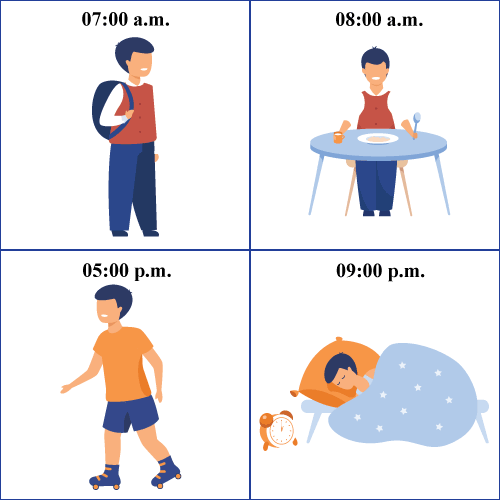## Time on a Number Line

Time can be represented in a number line as shown below.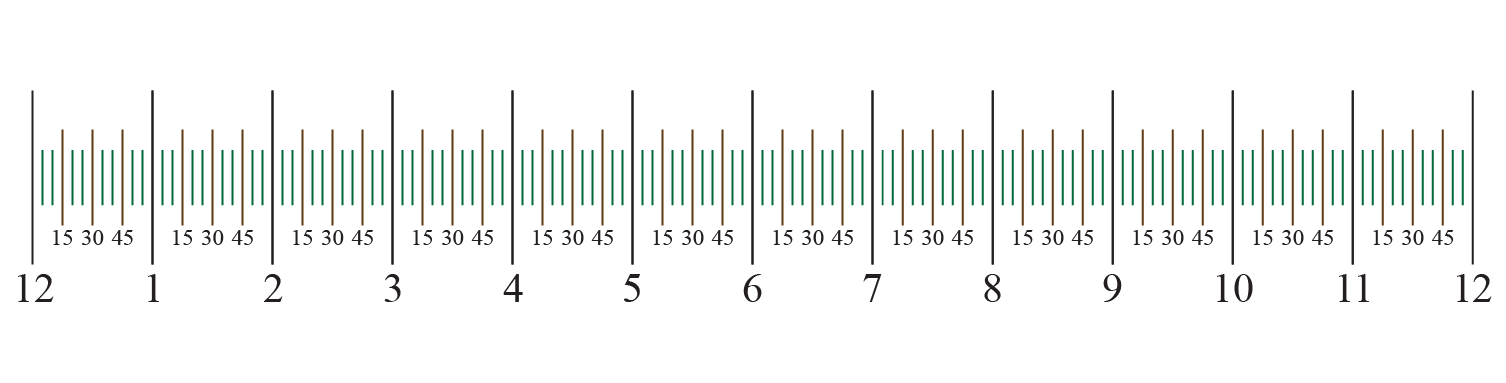This time number line shows 12 hours with the black line displaying every hour from 12 a.m. to 12 p.m.

Here, the brown lines denote the 15th, 30th, and the 45th minute of an hour.

The green line denotes the 5th, 10th, 20th, 25th, 35th, 40th, 50th, 55th minute.

This is one of the representations of timeline.

Time number lines are used to calculate how much time has elapsed between two given times.

## The Concept of Time Zones

In some parts of the world we define time based on time zones.

As the Earth rotates on its axis, we experience day and light. When a part of the Earth receives sunlight, it experiences day and the other parts experience night. This means that our lunch time may be someone else's sleep time since they live in that part of the earth where there is night.

Day-light saving is a practice of resetting clocks ahead by an hour or behind by an hour.

It is done in some parts of the world to make better use of the day time.

## Telling Time

Whenever someone asks the question - "What is the time right now"? We all look into a watch or a clock and say it is "X hours and Y minutes", In this section we will learn about time measurement.

Here 'X' (the hours) are usuallly expressed between 1 and 12 and 'Y' (the minutes) are expressed between 00 to 59.

There is also a third component here. It is called 'seconds'.

An hour consists of 60 minutes and a minute consists of 60 seconds. We will learn about various time unit conversion from hours to minutes, months to weeks and days.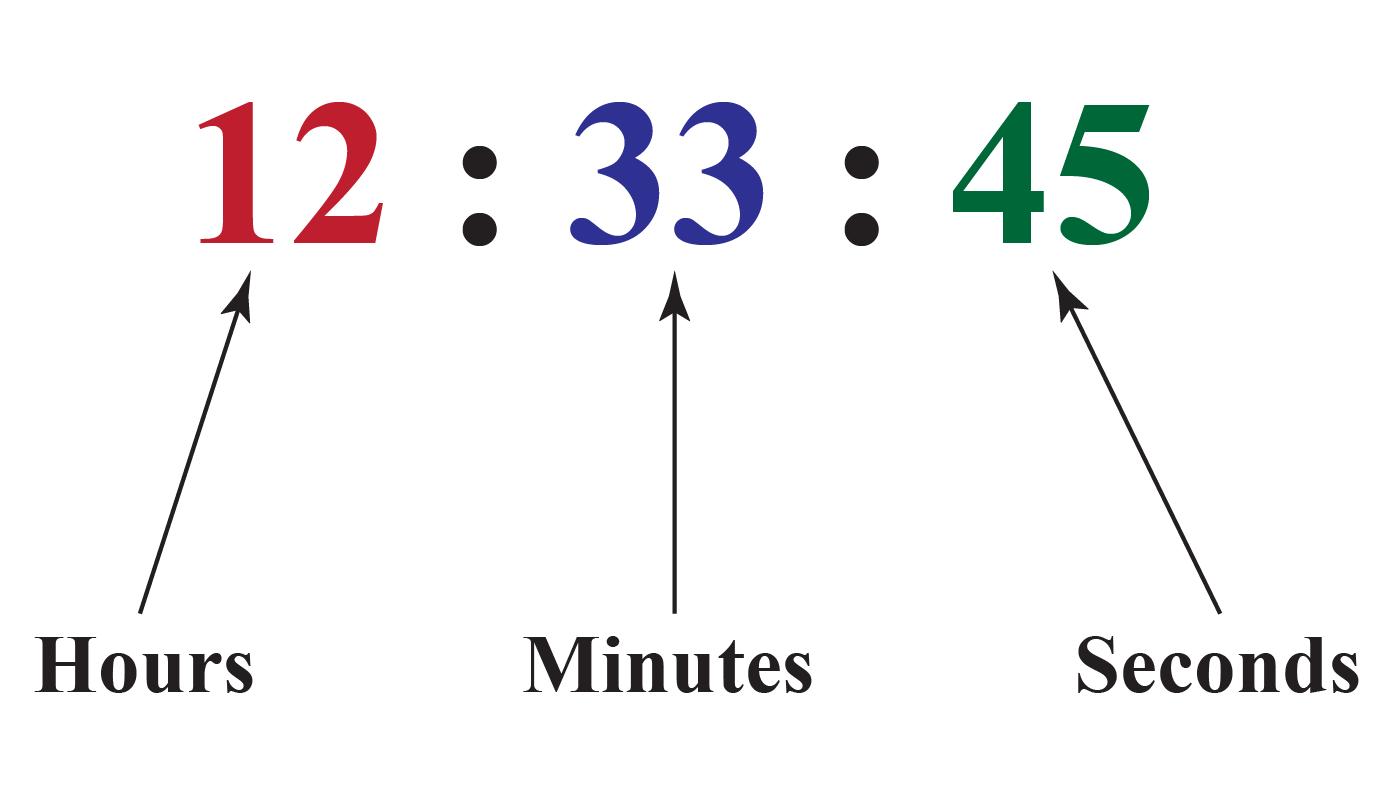### How Cuemath Students Learn Time?

The concept of time is told right from early childhood and Cuemath introduces this topic for kids since this is an important mathematical concept and they need to learn about this in relation to the different parts of the day. We need to know how to read time in a 12-hour and a 24-hour format too. It's about learning a valuable skill and understanding its various uses. Measurement of time in math can be done using clocks.

"Please be there on time" is one of the frequent phrases that we come across. Managing time is also an essential quality to organize our work and be successful. In order to be punctual and manage time well, we need to have a good understanding of time. Now let us move to the most important concept of reading time from a clock.

### Reading Time From a Clock

Reading time from a clock is an easy skill that comes with practice. Let us start from the largest unit of time which is an 'hour'.

A day has 24 hours but a clock has numbers only from 1 to 12 written on it. This means that there will be two rounds of 12 hours in a day.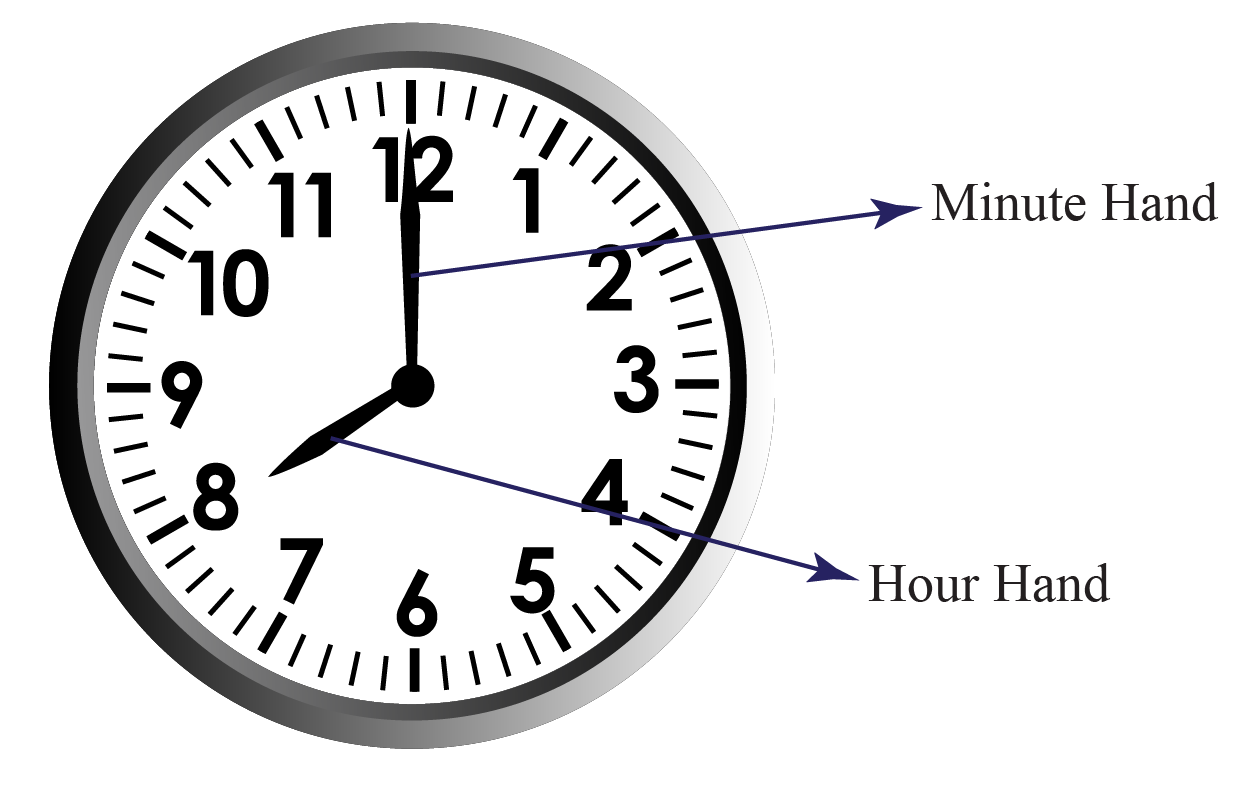A clock has 12 sections. Each number denotes an hour. There are two hands. The longer needle is the minute hand and the shorter needle is the hour hand. Each hand points to a number, and the combination of the hour hand and minute hand together give us the time.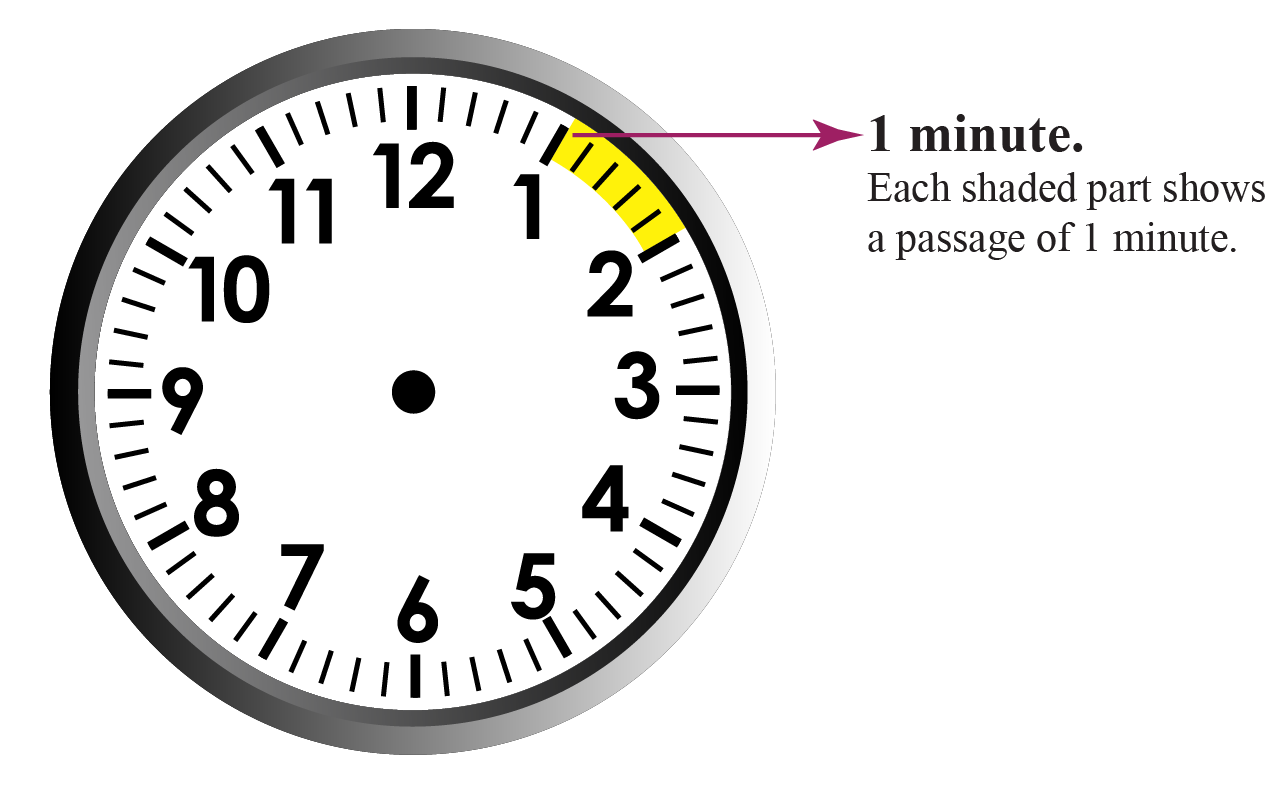The tiny lines between every number denote a minute. There are 5 minutes between any two numbers of the clock. The minute starts with 0 at number 12 and the count goes till 59.

Let us understand this with an example. Look at the clock shown below.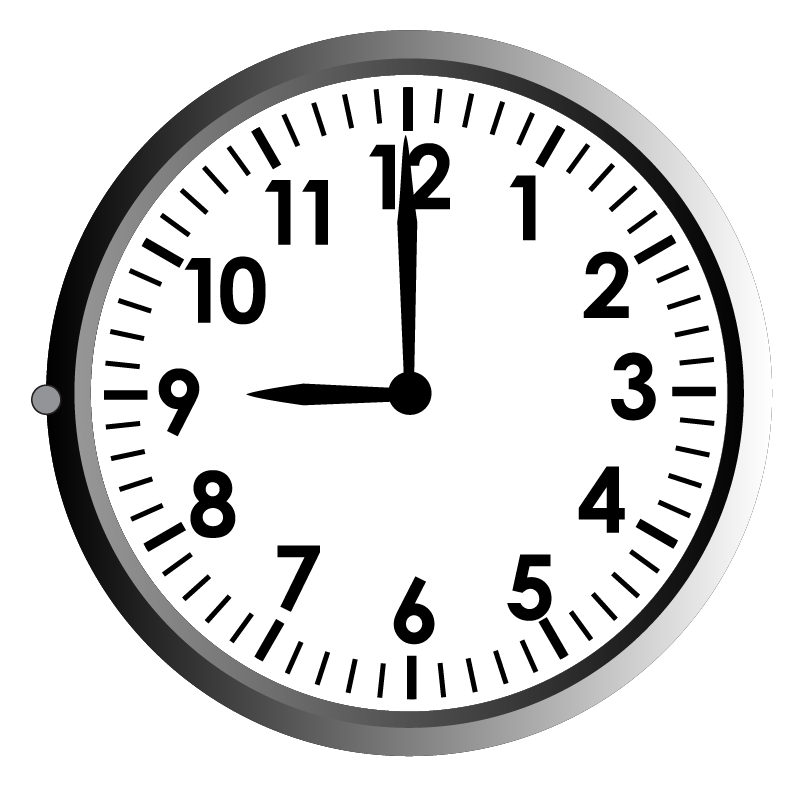The hour hand points to 9 and the minute hand points to 12. So, the time is 09:00 or 9 'o clock.Let us say that the hour hand started at 1 and the minute hand is at 12, which means the time is 1 'o clock. Now as the minute hand starts moving, the hour hand also progresses from 1 to 2. Everytime the minute hand reaches the next number starting from 12, it means 5 minutes have passed. So, in an hour the minute hand makes twelve 5 minutes move, which denotes that there are (12 x 5)  or 60 minutes in an hour.

The movement of the hour hand from one number to another marks the completion of an hour.

Some clocks have a third hand which is slightly thinner than the minute hand to denote the number of seconds passed in a minute.

Time measurement for 15 minutes, 30 minutes and 45 minutes can be represented as follows. They are:

Quarter to -

This means there are 15 minutes remaining to complete the given hour. For example, 05:45 means, there are 15 minutes to reach 6:00. This is read as "quarter to six".

Quarter past -

This means 15 minutes have passed after the hour has started. For example, 07:15 means, 15 minutes have passed after 7:00. This is read as "quarter past seven".

Half past -

This means 30 minutes have passed after the hour has started. For example, 08:30 means, 30 minutes have passed after 8:00. This is read as "half past eight".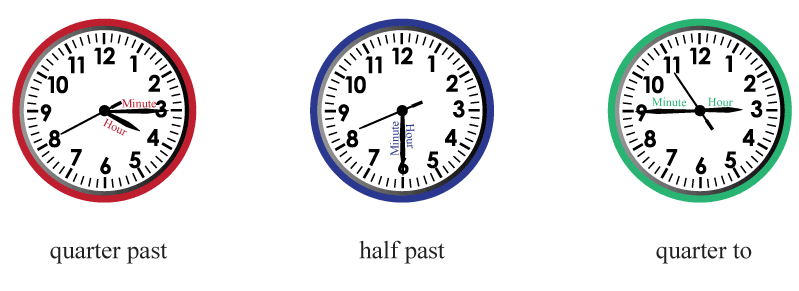### A.M. and P.M.

Time from 12:00 in the midnight to 11:59 in the morning is referred to as 'a.m'.

Time from 12:00 in the afternoon to late night 11:59 is referred to as 'p.m'.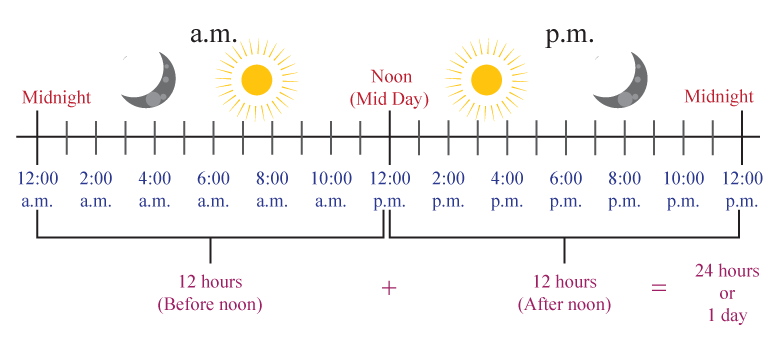### 12-hour and 24-hour clock

In the 12-hour time format, time is expressed with a.m or p.m.

8:20 in the morning can be told as 8:20 a.m. The same time in the evening is 8:20 p.m.

In the 24-hour time format, 7:30 a.m. is written as 7:30 hours, whereas 7:30 p.m. is written as 19:30 hours. We get this by adding 12 to the hour number. However, the minute number remains the same.

### Weeks, Months and Year

There are seven days in a week. The days of the week are named after the celestial bodies the Sun, the Moon, Mercury, Venus, Mars, Jupiter, Saturn.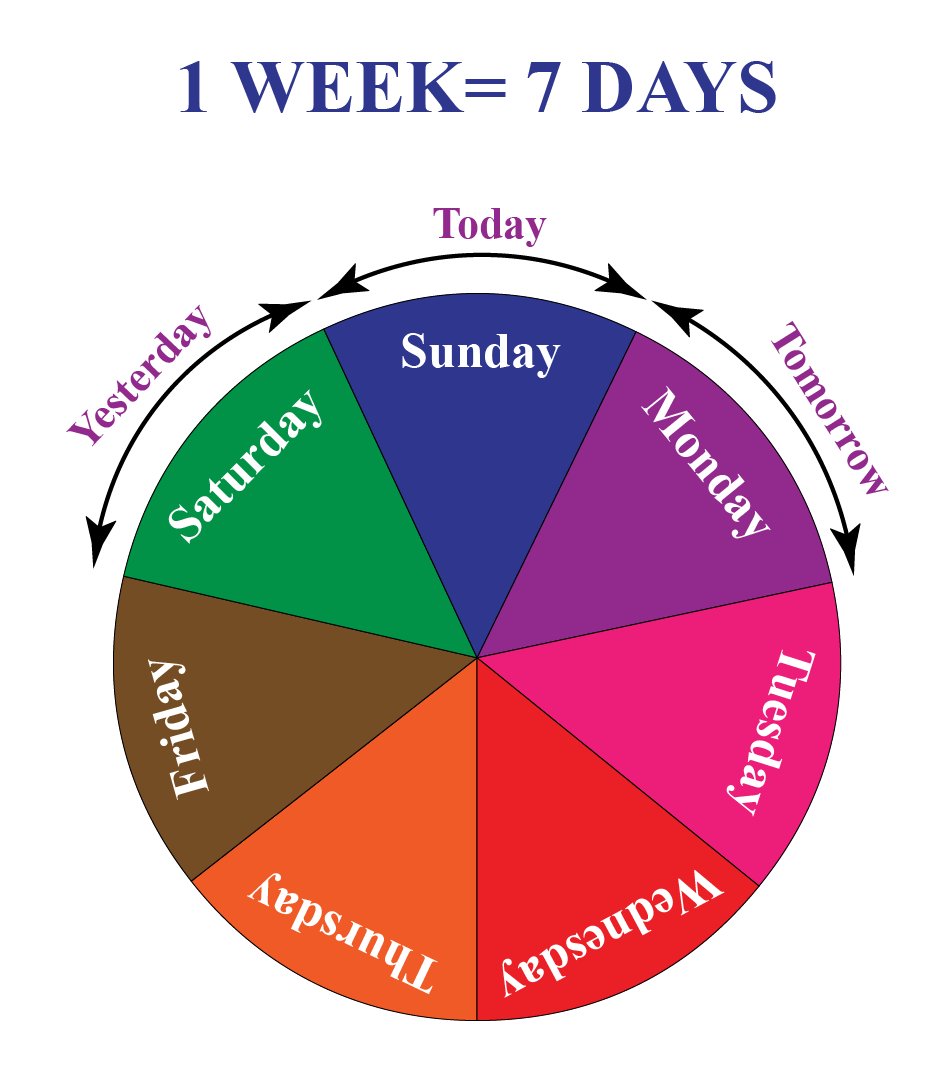Do you know how many weeks are there in a month? Every month has 4 full weeks for sure. Although a few months have more than 4 weeks. The extra days do not make up a week.

A month has 30 or 31 days. The month of february has 28 days for an ordinary year and 29 days for a leap year. A leap year comes once in every four years.

Month Days Weeks
January 31 4 weeks +  3 days
February

28 days (ordinary year)

29 days (leap year)

4 weeks + 1 day

March 31 days 4 weeks + 3 days
April 30 days 4 weeks + 2 days
May 31 days 4 weeks + 3  days
June 30 days 4 weeks +  2 days
July 31 days 4 weeks +  3 days
August 31 days 4 weeks + 3 days
September 30 days 4 weeks +  2 days
October 31 days 4 weeks +  3 days
November 30 days 4 weeks + 2 days
December 31 days 4  weeks +  3 days

The Gregorian Calendar is the most widely used calendar in which there are 365 days in an ordinary year and 366 days in a leap year, since there are 29 days in February.

To summarize,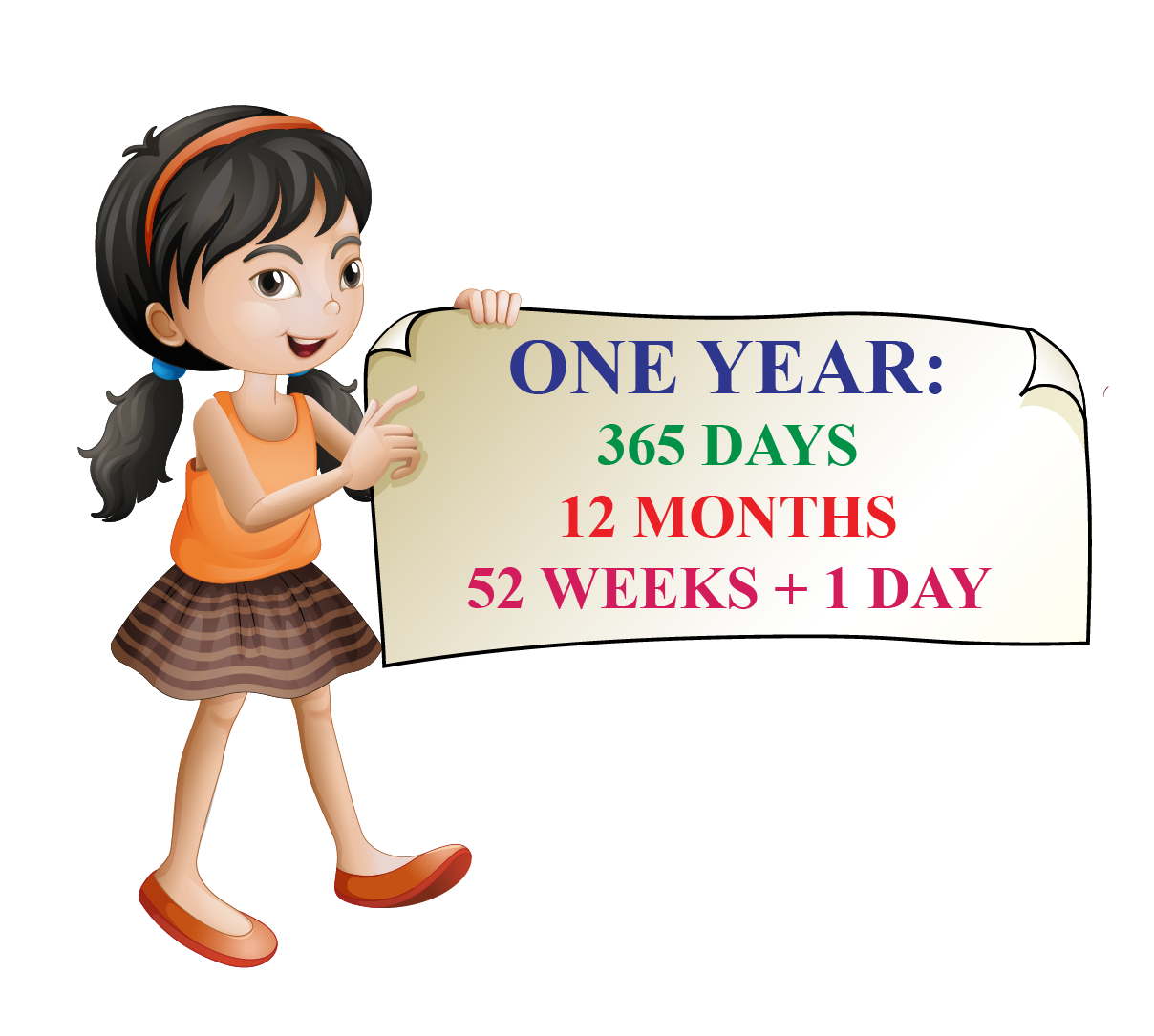Having told about the leap year, how do we check if a year is leap or not? Here you go!

Divide the given year by 4 days. If the division leaves 0 days as the remainder, then it is a leap year. Otherwise it is an ordinary year.

There is an exception for century years like 700,800,1700,1900,2000 days for which we divide the year by  400.

### 1. What is the SI unit of time?

The S.I. unit of time is 'Seconds' represented by the symbol 's'.

### 2. What is the smallest unit of time?

A zepto second is the smallest time unit. It is the trillionth of a billionth of a second.

### 3. What is the base unit of time?

'Second' is the base unit of time.

### 4. Why is it important to measure time?

Time is a very important measurement which helps us fix the duration or length of any event, specify when an event will start and end and also compare the duration of any two events.

### 5. Convert 1000 minutes to hour, 1000 seconds to minutes, 180 minutes to hours.

1000 minutes is equal to 16.66 hours, 1000 seconds is equal to 16.66 minutes, 180 minutes is equal to 3 hours.

More Important Topics
Numbers
Algebra
Geometry
Measurement
Money
Data
Trigonometry
Calculus